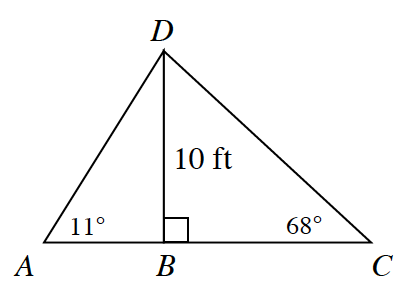### Home > GC > Chapter 4 > Lesson 4.1.2 > Problem4-20

4-20.

Examine the figure at right, which is not drawn to scale. Which is longer, $\overline{AB}$ or $\overline{BC}$? Explain your answer.By the slope ratio for $11º$$\frac{10}{AB}=\frac{1}{5}$

By the slope ratio for $68º$$\frac{10}{BC}=\frac{5}{2}$

Given the above information, solve for $\overline{AB}$ and $\overline{BC}$ to determine which is longer.